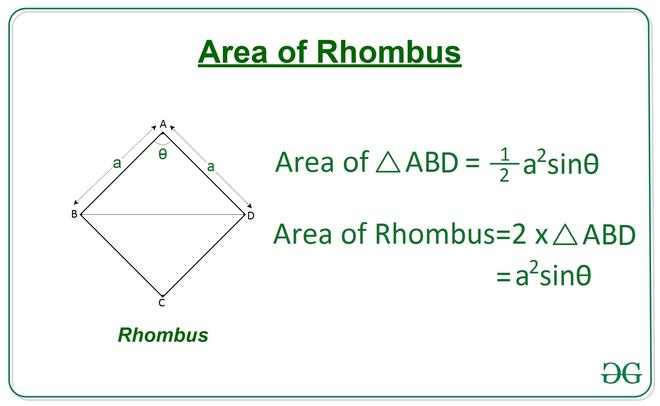# Find the area of rhombus from given Angle and Side length

Given two integers A and X, denoting the length of a side of a rhombus and an angle respectively, the task is to find the area of the rhombus.

A rhombus is a quadrilateral having 4 sides of equal length, in which both the opposite sides are parallel, and opposite angles are equal.

Examples:

Input: A = 4, X = 60
Output: 13.86

Input: A = 4, X = 30
Output: 8.0

Approach:For a Rhombus ABCD having the length of a side a and an angle x, the area of triangle ABD can be calculated using Side-Angle-Side property of triangle by the following equation:

Area of Triangle ABD = 1/2 (a2) sin x
Area of Rhombus ABCD will be double the area of ABD triangle.

Therefore, Area of Rhombus ABCD = (a2) sin xBelow is the implementation of the above approach:

## C++

 `// C++ Program to calculate` `// area of rhombus from given` `// angle and side length` `#include ` `using` `namespace` `std;`   `#define RADIAN 0.01745329252` `// Function to return the area of rhombus` `// using one angle and side.` `float` `Area_of_Rhombus(``int` `a, ``int` `theta)` `{` `    ``float` `area = (a * a) * ``sin``((RADIAN * theta));` `    ``return` `area;` `}`   `// Driver Code` `int` `main()` `{` `    ``int` `a = 4;` `    ``int` `theta = 60;`   `    ``// Function Call` `    ``float` `ans = Area_of_Rhombus(a, theta);`   `    ``// Print the final answer` `    ``printf``(``"%0.2f"``, ans);` `    ``return` `0;` `}`   `// This code is contributed by Rajput-Ji`

## Java

 `// Java Program to calculate` `// area of rhombus from given` `// angle and side length` `class` `GFG{`   `static` `final` `double` `RADIAN = ``0.01745329252``;` `  `  `// Function to return the area of rhombus` `// using one angle and side.` `static` `double` `Area_of_Rhombus(``int` `a, ``int` `theta)` `{` `    ``double` `area = (a * a) * Math.sin((RADIAN * theta));` `    ``return` `area;` `}`   `// Driver Code` `public` `static` `void` `main(String[] args)` `{` `    ``int` `a = ``4``;` `    ``int` `theta = ``60``;`   `    ``// Function Call` `    ``double` `ans = Area_of_Rhombus(a, theta);`   `    ``// Print the final answer` `    ``System.out.printf(``"%.2f"``, ans);` `}` `}`   `// This code is contributed by Rajput-Ji`

## Python3

 `# Python3 Program to calculate ` `# area of rhombus from given ` `# angle and side length` `  `  `import` `math  ` `  `  `# Function to return the area of rhombus ` `# using one angle and side.  ` `def` `Area_of_Rhombus(a, theta):  ` `  `  `    ``area ``=` `(a``*``*``2``) ``*` `math.sin(math.radians(theta))` `  `  `    ``return` `area  ` `  `  `# Driver Code  ` `a ``=` `4` `theta ``=` `60` `  `  `# Function Call  ` `ans ``=` `Area_of_Rhombus(a, theta)  ` `  `  `# Print the final answer` `print``(``round``(ans, ``2``)) `

## C#

 `// C# Program to calculate` `// area of rhombus from given` `// angle and side length` `using` `System;` `class` `GFG{`   `static` `readonly` `double` `RADIAN = 0.01745329252;` `  `  `// Function to return the area of rhombus` `// using one angle and side.` `static` `double` `Area_of_Rhombus(``int` `a, ``int` `theta)` `{` `    ``double` `area = (a * a) * Math.Sin((RADIAN * theta));` `    ``return` `area;` `}`   `// Driver Code` `public` `static` `void` `Main(String[] args)` `{` `    ``int` `a = 4;` `    ``int` `theta = 60;`   `    ``// Function Call` `    ``double` `ans = Area_of_Rhombus(a, theta);`   `    ``// Print the readonly answer` `    ``Console.Write(``"{0:F2}"``, ans);` `}` `}`   `// This code is contributed by Rajput-Ji`

Output:

```13.86

```

Time Complexity: O(1)
Auxiliary Space: O(1)

Attention reader! Don’t stop learning now. Get hold of all the important DSA concepts with the DSA Self Paced Course at a student-friendly price and become industry ready.

My Personal Notes arrow_drop_upCheck out this Author's contributed articles.

If you like GeeksforGeeks and would like to contribute, you can also write an article using contribute.geeksforgeeks.org or mail your article to contribute@geeksforgeeks.org. See your article appearing on the GeeksforGeeks main page and help other Geeks.

Please Improve this article if you find anything incorrect by clicking on the "Improve Article" button below.

Improved By : Rajput-Ji